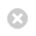Close

Mathematics

Home# Mathematical Physics

### Continuum mechanics

Continuum mechanics is a branch of applied mathematics which studied the kinematics and dynamics of materials which are modelled as continuous mass distributions rather than a set of discrete particles bound together. The field of Cosserat elasticity or micropolar elasticity is of particular interest to our group. It shows some surprising similarities with general relativity. For instance, microrotations can be described using concepts from differential geometry, in particular the torsion tensor. It turns out that the action of microrotations looks like a three-dimensional version of the action of teleparallel gravity.

### Integrable systems

Integrable systems are often described by non-linear ordinary differential equations or non-linear partial differential equations for which it is possible to find exact solutions. Two well-known examples are the Korteweg–de Vries equation which can be used to model waves on shallow water waves and the Sine-Gordon equation which has soliton solutions. Other systems of interest are discrete systems. Work at UCL aims at understanding under which conditions a system of equations is integrable.

### General Relativity and Cosmology

General Relativity and Cosmology are two exciting topics of current research in theoretical physics. Our group focusses on two aspects: modified gravity and dynamical systems in cosmology. A variety of modified gravity theories have been analysed and it was found that some of these models satisfy cosmological and local gravity constraints and are practically indistinguishable from the ΛCDM model, at least at the background level. There are many generalisations and modifications of the Einstein-Hilbert action. The use of dynamical systems in cosmology allows the study of very generic cosmological models which can then be tested against observational data.

### Many-body Quantum Physics

Strongly-correlated matter abounds in a number of exotic phenomena that have perplexed physicists and mathematicians alike. These include superconductivity, superfluidity or fictious electric and magnetic charges. Strong correlation between individual particles requires novel mathematical modelling that can involve quantum field theories, renormalisation group methods and matrix-product states to name but a few. Work at UCL utilises these to explore a variety of many-body quantum phenomena. Of particular current interest are the interplay of Kondo screening, magnetism and superconductivity, the dynamics of quantum spin liquids, and the processes of thermalisation and localisation.